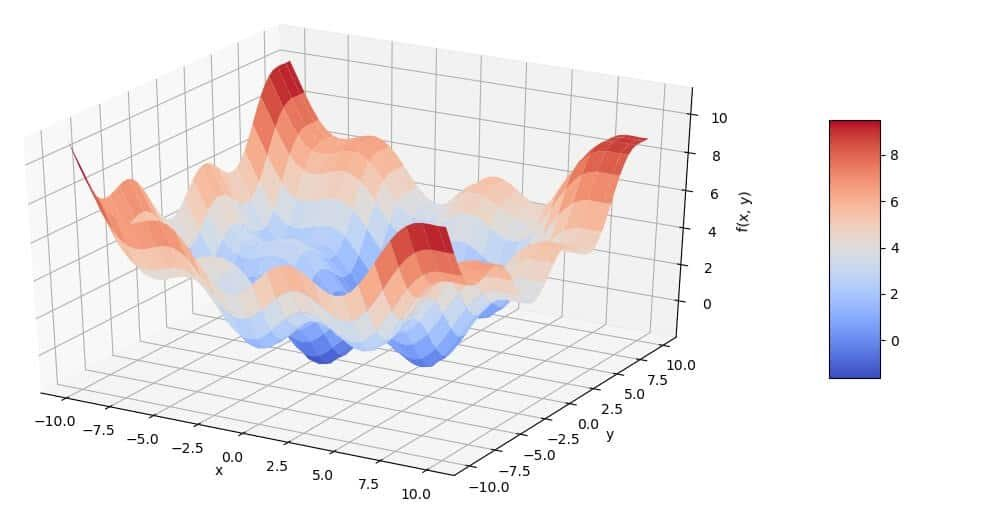Convex optimization is a field of mathematical optimization that studies the problem of minimizing convex functions over convex sets.

Convex optimization has applications in a wide range of disciplines, such as automatic control systems, estimation and signal processing, communications and networks, electronic circuit design, data analysis and modeling, finance, statistics, etc.

With recent advancements in computing and optimization algorithms, convex programming is nearly as straightforward as linear programming.

In finance and economics, convex optimization plays an important role. Examples are the calibration of option pricing models to market data or the optimization of an agent’s utility.

Previously, we wrote about Monte Carlo Simulation and if you haven’t read yet, we strongly suggest you do so.

In today’s finance with Python article, we are going to show you how to optimize a function using Global and Local optimization.

### Convex optimization functions using Python programming language

Copy to Clipboard

Above is our starting example. This example is inspired by the book Python for Finance: Analyze Big Financial Data:

We modified it and translated it to Python 3, since it is written in Python 2, and the code was not quite working, so we’ve had to fix tons of stuff, but what you see here works 100%.

The result of the code above is in Figure 1.Figure 1: Plotting the start function

From Figure 1 we can see that the function already has multiple local minima, but the global minima cannot be confirmed from the visualization we’ve got.

### Local Optimization

Copy to Clipboard

This is the code we’ve implemented to find the local minimum on the whole of the interval, which is the lowest minimum of them all.

## Conclusion

Convex optimization is very important to be understood by every engineer since it’s a base for some more complicated and effective optimization problems. It has a lot of algorithms that you might find helpful so you can search for them and try to implement them.